Electrics Index

ElectroMagnetism

Introduction
Basic Notes on Magnets

If a permanent magnet is suspended, so that it is free to swing in a horizontal plane, it will take up such a position that one end will point towards the north pole.  This end is said to be the north-seeking end of the magnet.  The other end of the magnet is called the south seeking end.  Therefore the earths north pole is actually its south magnetic pole

When considering a magnetic field which is composed of imaginary lines of magnetic flux, by common convention the direction of the magnetic field is that which a north-seeking end of a bar magnet points.

Lines of magnetic flux form closed loops.  They emerge from the N-pole end of a magnet pass through the surrounding space and into the S-pole end of the magnet.  They are then assumed to pass along the magnet to emerge again at the N-pole.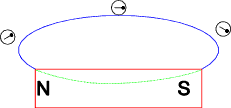Lines of magnetic flux never intersect.

Lines of magnetic flux are always trying to shorten themselves.  Therefore if two magnets are brought together with the north end of one close to the south end of the other, the lines of flux will arrange themselves to cause the magnets to be attracted.  See figure below.

Lines of magnetic flux which are parallel and in the same direction repel one another.  See figure below.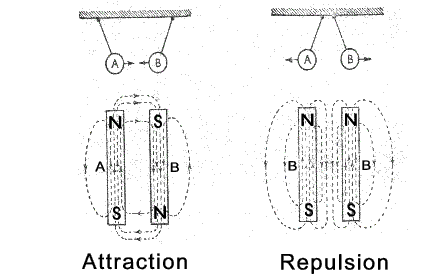Nomenclature

I = current flow (amperes)
i = instantaneous current flow (amperes)
E = e.m.f. (volt)
V = potential difference (volt)
e = instantaneous e.m.f. (volt)
v = instantaneous potential difference (volt)
l = length (m)
B = flux density (Teslas = webers/m2)
F = force (N)
H = magnetic field strength (A -turns/m)
Φ = flux (webers)
Ψ = flux linkages (weber -turns)
μo = permeability of free space (H /m)
μr = relative permeability
μ = absolute permeability =μo.μr

Conversions
In older textbooks values are given in (CGS) units .  Below are a small number of useful conversions .

 Symbol Description One unit Is equal To SI unit CGS unit B Magnetic Flux Density Tesla (= 1 Wb / m2) 104 Gauss F Magnetomotive force A (= amp  turn ) 1,25664 gilbert H Magnetic Field Intensity A / m (= amp  turn / m ) 0,012566 oersted Φ Magnetic Flux Wb 108 maxwells

Magnetic Induction

If a soft iron block is located within a magnetic field the lines of flux will pass through the iron block and it will become a temporary magnet.  The iron block is magnetised as a result of magnetic induction.  The figure below shows a U shaped permanent magnet with two soft iron blocks (A & B) attached to the magnet and a cylinderical block C located between A & B.   No lines of magnetic flux pass through the hole in the cylinderical block and any object placed in this hole would be screened from the magnetic field.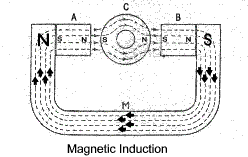Magnetic Field Due to an Electric Current

When a conductor carries an electric current a magnetic field is produced around that conductor.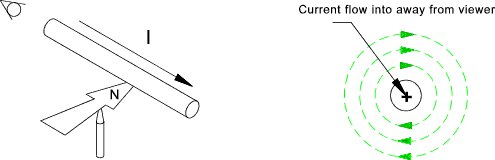When a conductor is formed into a solenoid the resulting magnetic field formed is as shown in the figure below. The relationship between the current flow around a solenoid and the magnetic field is identified using the right hand rule.."If the right hand grips the outside of a coil with the fingers in the direction of the current flow the thumb points in the direction of the magnetic field in the centre of the solenoid."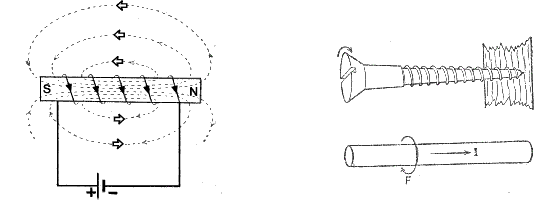Magnetic Force on a Conductor in a magnetic field

When a conductor carrying an electric current is located in a magnetic field the lines of flux generated by the current are opposed to the fixed field at one side and they combine with the fixed field at the other side.  The result is that there is force on the conductor as shown in the figure below..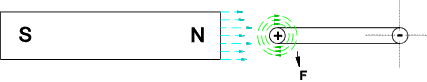>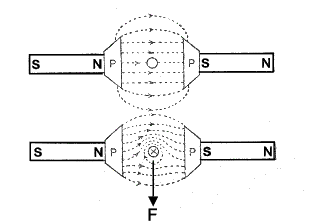The force on a conductor in a magnetic field is related to the current and is also related to the strength of the magnetic field.

Flemings Left Hand Rule.

This is used to identify the relative directions of the field, current and motion (Thrust) when a current flows in a conductor in a magnetic field........

If the Thumb, first finger and second fingers of the left hand are held pointing at right angles to each other and the first finger is pointing along the magnetic field and the second finger points in the direction of current flow then the thumb points in the direction which the conductor will tend to move.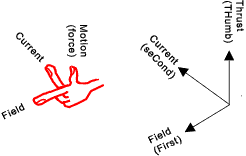Applying this to an electric motor..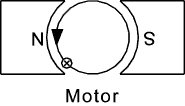The definition of flux density (Flux Density) is such that the force on a conductor of length l in a magnetic field of flux density B webers when the current flow in the conductor is I amperes is

F (newtons) = I.l.B

Electromagnetic Induction

In 1831 Michael Faraday discovered that if a conductor moves relative to a field of magnetic flux a current flow is induced in the conductor.   The magnitude of the induced current is related to the strength of the magnetic field and to the velocity of relative motion.

Consider a negative charge (-q )moving towards the left in a downward direction magnetic field which is equivalent to a current ( I )in the opposite direction .  Both experience a force as shown in the figure below.  In the same way a positive charge (+q ) moving to the left which is equivalent to a current I moving in the same direction will experience a force in the opposite direction.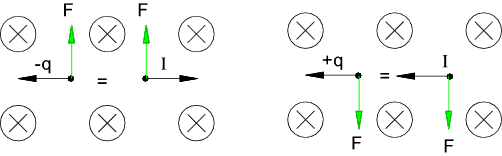Now consider a conductor moving towards the left at a velocity of v.  The electrons will experience a force F tending to move them up leaving positive ions at the other end of the conductor.   This movement will continue until the force F moving the charges is balanced by the resulting e.m.f opposing the motion.. ref, Def. Flux Density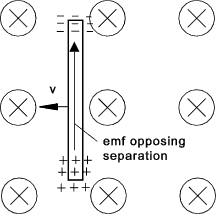If the length of the conductor is l then the work done in moving a unit charge Q along the conductor is

W = Blv This is equal to e.m.f along the conductor of...E = Blv
(A charge of 1 coulomb acquires or delivers an energy of 1 Joule in moving through a potential difference of 1 volt.)

In general the e.m.f generated in a conductor moving in an electric field is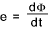1) While the magnetic flux linking with a circuit is changing, an e.m.f is induced in the circuit.

2) The magnitude of the induced e.m.f. at any instant is proportional to the rate at which the flux through the circuit is changing.

Combined these two laws state...While the magnetic flux through a circuit is changing , an e.m.f which is proportional to the rate of change of flux is induced in the circuit.

Lenz's Law is stated as follows.

"The direction of induced e.m.f is always such that it tends to set up a current opposing the motion or the change of flux responsible for inducing that e.m.f".

Flemings Right Hand Rule

This identifies the relative directions of the induced current,field and motions..

If the thumb , first finger, and second finger are all held at right angles to each other and if the first finger points along the field and the thumb in the direction of motion then the second finger shows the direction of induced current or E.M.F.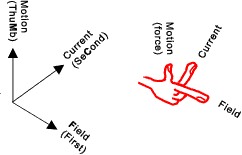Applying this to an generator..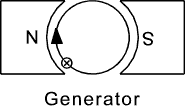Magnitude and Direction of Induced Current

A magnet moving into a coil induces current flow as shown.   A similar condition occurs with two adjacent coils when a current flow is initiated, as shown in red, in one of the coils.  The magnetic field (shown in red) as it increases is similar to a magnet moving into the second coil induces an e.m.f resulting in a current flow flow as shown in green.   This induced current flow results in a magnetic flux opposing the original flux as shown (in green).  When the current flow in the primary circuit stabilises the flux is constant and the induced e.m.f in the secondary circuit becomes zero.  If the switch is now opened the flux will collapse causing a current flow in the second circuit which tends to oppose the collapsing field.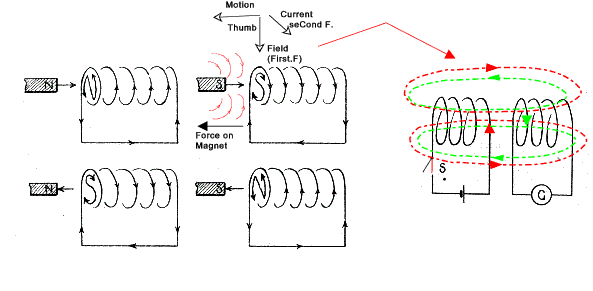Let the magnetic flux through a coil of N turns be increased by Φ webers in t seconds as a result of the relative movement of a fixed magnet (or due to the generation of a field by initiating current flow in an adjacent coil).  Since each line of flux cuts each turn of the coil then the average e.m.f induced in each coil is Φ /t volts.  Following lenz's law the current resulting from this e.m.f tends to prevent the changing flux - it tends to set up an opposing flux.  The result of this interaction is that the coil tends to oppose the movement of the magnet.

The flux linkages NΦ are given the symbol Ψ .   The average e.m.f induced in the coil is NΦ /t volts which is defined as the average rate of change of flux linkages per second (weber -turns).  In general if the magnitic flux through a coil increases by dφ in dt seconds the e.m.f induced in the coil is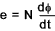From the above it is is possible to define a weber as the magnetic flux which linking a circuit of one turn induces an e.m.f of 1 V when the flux is reduced to zero at a uniform rate in 1 second.

Considering two coils (C1 & C2) located such that the flux generated in one coil links the second coil as shown in the figure below.
If C2 is initially open circuit such that no current can flow in C2.   Then if when the switch is closed the flux in the ring resulting from the current flow in C1 increases by Φ webers in t seconds.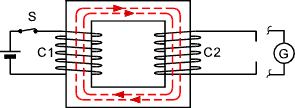If the connected coil C1 has N 1 turns then the average e.m.f induced is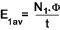The direction of this e.m.f. (according to Lenz's law) is such that it tends to oppose the growth of the current.

Now if coil C2 with N 2 turns is connected to allow current to flow and the switch is closed to allow current to flow.  All of the resulting flux produced by coil C1 links C2 and the average e.m.f induced in C2 is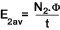Again the e.m.f circulates a current which tends to induce a flux which opposes the flux that is induced by the rising current in coil C1...

Magnetic Circuits

The complete close path followed by any group of magnetic flux lines is called the magnetic circuit.  In the sketch below the magnetic circuit is a steel toroidal ring with a coil which induces the flux when current flows.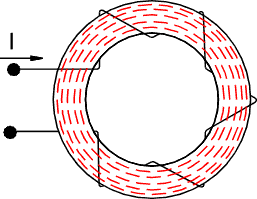A magnetic circuit has similar relationships to an electric circuit.   In an electric circuit the current flows due to the electromotive force (e.m.f) while in a magnetic circuit the flux is due to the magnetomotive force m.m.f.   The m.m.f. is proportional to the current flowing and the number of turns and is called the Ampere Turns.   Dimensionally it is simply Amperes because turns is not really a dimension quantity.   The m.m.f is given the symbol F.

If as in the figure above I amperes flow through N turns the m.m.f. is IN amperes (often called ampere turns).

If the flux circuit is of uniform section and is homogeneous then the m.m.f per metre length of circuit is called the magnetic field strength and is given the symbol H.  If the mean length of the circuit in the above figure is l then the magentic field strength H = IN/l.  The units for magnetic field strength are amperes /metres (often called ampere turns /metre)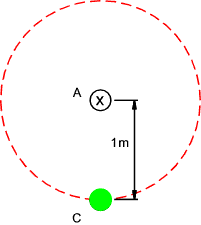Consider a single conductor A carrying a current of 1 Ampere as shown with only the effects of the current flow down being considered (the return flow path being some distance away).   The magnetic field strength at a radius of 1 m is 1 ampere(turn) and the length of the field is 2π.metres.  The magnetic field strength at 1m is therefore 1/(2.π) amperes(turns) per metre.

If the flux density in the region of the line of flux shown is B Teslas the the force per metre length on a conductor C carrying a current of 1 ampere located parallel to A at 1 metre distance is B(T).1 (m).1 (A) = B newtons.

Note..The ampere is defined as the constant current which when flowing through two infinitely long parallel wires 1 m apart would produce in vacua a force of 2 x 10 -7 N/m on each conductor

Therefore the flux density at 1m radius from a conductor carrying 1 A is B = 2 x 10-7 T. Hence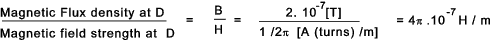The ratio B/H for a vacuum is called the permeability of free space and is given the symbol μo with units henry per metres and is virtually the same for a vacuum , air , and other non-magnetic materials such as wood, oil, copper etc

The magnetic field strength for any non-magnetic materials is H = B / μo = B / (4π. 10-7)) amperes per metre

Note : J.C.Maxwell discovered in 1865 that 1/Sqrt(μo.εo) = 2,998.108 m/s = c = The speed of light

The magnetic flux resulting from a current flowing in a coil can be increased if the medium containing the flux is a magnetic medium such as steel or a ferromagnetic material containing elements such a iron, cobalt, nickel, gadolinium etc.  The ratio of the flux density of produced in a material compared to the flux density produced in a vacuum is called the relative flux permeability (μr).

The absolute permeability of a material in which there is a magnetic circuit μ = μr. μo).  For a magnetic circuit.

μ = B/H

μr for non magnetic materials is generally about 1.   For ferromagnetic materials iron, steel etc μr varies significantly as H increases.  To illustrate this point ref to figure below showing a very very approximate B/H curves for different ferromagnetic materials.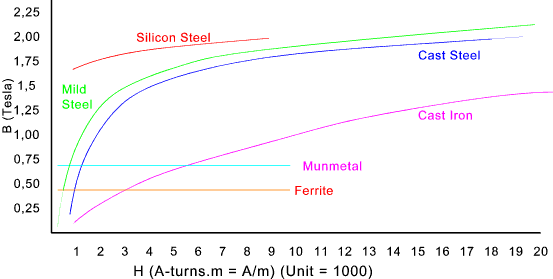Hysterisis

When considering ferromagnetic materials in electromagnets subject to alternating currents such as in electric motors and transformers it should be noted that the magnetic flux does not move up and down a single curve as the initiating electrical current rises and falls.  In practice the magnetic flux follows a curve approximated by the figure below.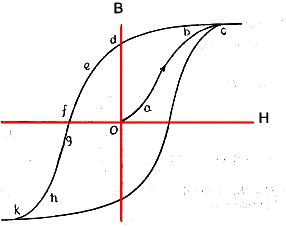Initially the ferromagnetic material is unmagnetised at 0 then, as H increases, the flux (B) follows the path a-b-c.  At c the material is effectively saturated.  Now if H is reduced to zero degree of magetism moves along the curve to d.  Although the magnetising force has reduced to zero the ferromagnetic material has retained a most of its magnetism.

Reversal of H causes the flux to move along the curve along d-e-f k.   The material has now been completely demagnetized and remagnetised to the saturation level in the reverse direction.   If H then increases back to its maximum value in the positive direction the flux moves from k to c.

This curve is a hysteresis curve and the area enclosed within the curve is effectively energy losses.  When considering electric machine performances this energy loss is called hysterisis loss.

Analogy between Magnetic and Electrical Circuits

 Electrical Variable Magnetic Variable Magnetic Units Voltage (V) Magnetomotive force F = N.I Amp-turns Current (I) Magnetic flux � webers Resistance (R = ohm ) = ρ. l / A Reluctance R = (1 / μ).l / A Amp-turns / Weber Current Density (J= amperes / m 2) Magnetic flux density B Wb/m2 = teslas T Electric Field (E= volts / m) Magnetic field intensity H Amp-turn/m

Comparison of Electromagnetic and Electrostatic Variable names and Symbols
 Electrostatics Electromagnetics Names Symbol Name Symbol Electric flux Q Magnetic flux Φ Electric flux density D Magnetic flux density B Electric field strength E Magnetic field strength H Electromotive force E Magnetomotive Force F Electric potential difference V Magnetic potential difference - Permittivity of free Space εo Permeability of free space μo Relative permittivity εr Relative permeability μ Absolute permittivity D/E Absolute permeability B/H

 Useful relevant Links NDT resource Centre- Magnetism... Clear detailed notes covering all relevant topics Magcraft Magnet University- Magnetism... A magnet supplier with a very informative website 5. Electromagnetism... Some fairly advanced notes on Electromagnetism Electromagnetism... Some advanced papers on related stuff Answers.com Electromagnetism ...A highly integrated set of look up notes abyss- Lecture Notes......A wide ranging set of notes very clear information transfer Basic Electric and Magnetic Circuits... A pdf download paper including a full set of notes Wikipedia-Eelctromagnetism wikipedia -Proving to be an excellent source of information

Electrics Index